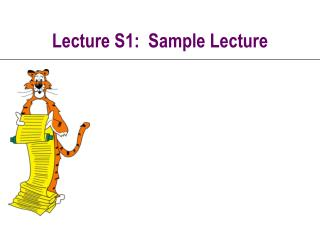DownloadDownload PresentationLecture S1: Sample Lecture

# Lecture S1: Sample Lecture

Télécharger la présentation## Lecture S1: Sample Lecture

- - - - - - - - - - - - - - - - - - - - - - - - - - - E N D - - - - - - - - - - - - - - - - - - - - - - - - - - -
##### Presentation Transcript

1. Lecture S1: Sample Lecture

2. Overview • Lecture T4: • What is an algorithm?Turing machine • Which problems can be solved on a computer?Not the halting problem. • Here’s a question for the student to fill in the answer. • Here’s the secret answer.

3. The Main Question P = NP NP P If P  NP If P = NP Kevin Wayne: all NP problems are solvable, unlike Halting problem • First level. • Second level. • third level • fourth level • Most important open problem in theoretical computer science. Also ranked #3 in mathematics (Smale).

4. While Loop Example #include <stdio.h> int main(void) { float x, y; printf(“ x f(x)\n”); x = 0.0; while (x < 2.0) { y = 2.0 - x*x*x; printf(“%4.1f %6.3f\n”, x, y); x = x + 0.1; } return 0; } table2.c • Print a table of values of function f(x) = 2 - x3. A second attempt. uses while loop

5. Anatomy of a While Loop x = 0.0; while (x < 2.0) { y = 2 - x*x*x; printf(“%f %f”, x, y); x = x + 0.1; } C code • Previous program repeats the same code over and over. • Repetitive code boring to write and hard to debug. • Use while loop to repeat code. x  0 y  2 - x3 print x, y x  x + 0.1 x < 2.0 true false

6. While Loop Example #include <stdio.h> int main(void) { float x, y; printf(“ x f(x)\n”); x = 0.0; while (x < 2.0) { y = 2.0 - x*x*x; printf(“%4.1f %6.3f\n”, x, y); x = x + 0.1; } return 0; } table2.c • Print a table of values of function f(x) = 2 - x3. A second attempt. uses while loop